### IMO Shortlist 1988 problem 8

Kvaliteta:
Avg: 0,0
Težina:
Avg: 0,0
Let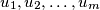$u_1, u_2, \ldots, u_m$ be$m$ vectors in the plane, each of length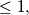$\leq 1,$ with zero sum. Show that one can arrange$u_1, u_2, \ldots, u_m$ as a sequence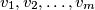$v_1, v_2, \ldots, v_m$ such that each partial sum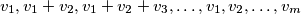$v_1, v_1 + v_2, v_1 + v_2 + v_3, \ldots, v_1, v_2, \ldots, v_m$ has length less than or equal to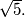$\sqrt {5}.$
Izvor: Međunarodna matematička olimpijada, shortlist 1988